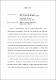## Magneto-optical response of WSe2 excitons in sub-Kelvin regimeInterest in correlated electron states in strongly correlated materials, such as transition metal dichalcogenides (TMDs), has been growing in recent years thanks to significant technological advancements in cryogenic quantum optics measurement techniques that allow for their experimental realization. Milli-kelvin temperatures are required to eliminate thermalization effects that otherwise mask the interesting correlated physics in these materials. In an effort to contribute to the understanding of these states, we developed an ultra-low temperature dilution refrigerator measurement system with free-space optical access that is able to achieve a base temperature of $<$30 milli-kelvin and apply a $\pm$12 T perpendicular magnetic field. We utilize a 100x cryogenic objective with 0.82 NA to make highly localized optical measurements on our samples. Our custom stage system allows for quick sample exchange and rapid characterization of our devices. TMDs have been shown to be suitable materials for probing new and interesting exciton physics. In monolayer form, their direct band gap in the visible range and high binding energies open the possibility to optically explore higher energy Rydberg states. As the understanding of the neutral exciton's Rydberg series has grown in recent years, interest has started to shift toward the less understood charged excitons. Using photoluminescence excitation (PLE), we sweep a laser's energy across the spectral range of the $n>1$ Rydberg exciton states and monitor the emission intensities of the 1$s$ excitons. Through this technique, we were able to measure the precise spectral profile and carrier density dependence of the 3$s$ neutral (X$_0^{3s}$), 2$s$ neutral (X$_0^{2s}$), and 2$s$ negatively charged (X$_-^{2s}$) excitons in monolayer WSe$_2$. By applying a perpendicular magnetic field to the sample, we were also able to measure the valley dependent Zeeman splitting of both X$_0^{2s}$ and X$_-^{2s}$ and extracted their corresponding g-factors.